## HOW MUCH DO YOU KNOW ABOUT POLYGONS?

Dear Students: Polygons are all around us. They help us understand our world and how to get around in it. Think about a stop sign. You probably already know that a stop sign has eight sides. Because we have become used to a stop sign having eight sides, we may think STOP! whenever we see an eight-sided polygon even if it doesn't have the word, STOP, painted on it.

How much do you know about polygons? Check out your knowledge by taking the Quickie Quiz below.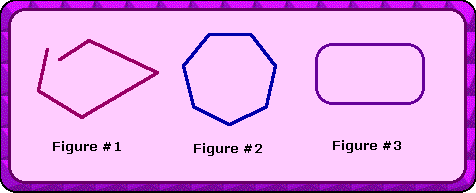Figure #1

Figure #2

Figure #3

Question 2: Which figure is a quadrilateral?Figure #1

Figure #2

Figure #3

Question 3: What is the name of this polygon?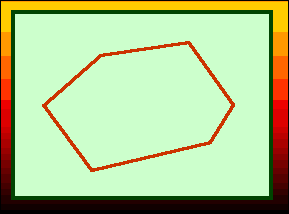pentagon

octagon

hexagon

Question 4: Which figure is a dodecagon?Figure #1

Figure #2

Figure #3

Question 5: Which figure is a type of parallelogram?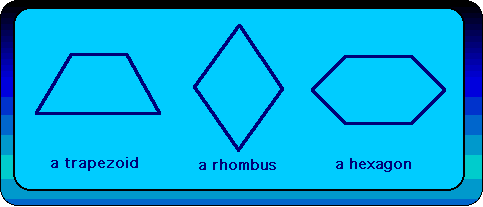Figure #1

Figure #2

Figure #3

Question 6: Which figure is a concave polygon?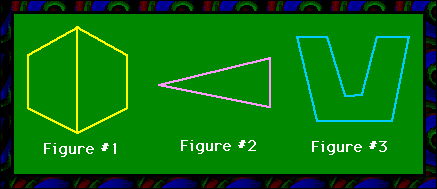Figure #1

Figure #2

Figure #3

Question 7: Which figure has seven sides?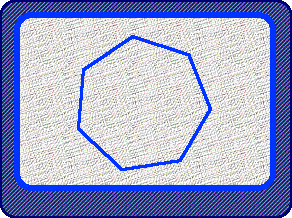A hexagon

A nonagon

A heptagon

Question 8: An n-gon has how many sides?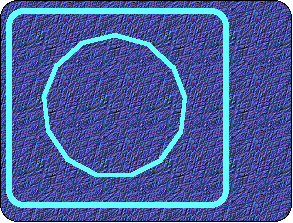n-sides

nine sides

no sides

Question 9: Which figure is a regular polygon?Figure #1

Figure #2

Figure #3

Question 10: Which description matches this figure?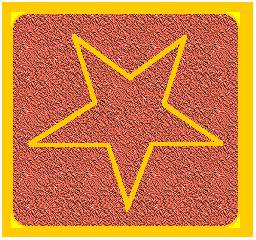Concave decagon

convex decagon

congruent decagon

SCORING

Score one point for each question that you got correct.

Score a Bonus Point if you took the time to read the answers and explanations.